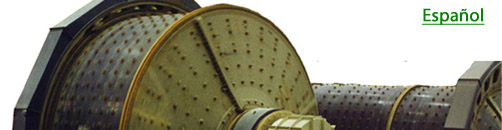expertise in mineral grinding systemsIssue No. 5                                                                                     February, 2014   What exactly is 'Work Index' and how can I make use of it?     'Work Index' is to measuring energy in size reduction what the 'meter' is to measuring distance. It is a standard measure that brings all size reduction circuits onto a common basis for comparison. It starts with Bond's Work Index equation:W is the work (energy) input per ton. F80 is the 80% passing size of the circuit feed, and P80 is the 80% passing size of the circuit product, in microns. For example, a grinding circuit is processing 450 t/h (of dry feed) with a mill drawing 3,150 kW (at the pinion). The circuit feed is 80% passing 2,500 um, and the circuit product is 80% passing 212 um.Then WIo, the "Operating Work Index" of this circuit, is calculated as follows:So for this circuit on this ore,We can now estimate the needed W if, for example, we accept a grind P80 of 250 um.So with the same mill power we can increase the tonnage to:Estimating the new production rate by changing one of the variables is just one of the ways you can use this equation. Note that if we make the feed size very large, the term 10/√F80 would approach a value of zero. If at the same time we make the P80 equal to 100 um, the term 10/√P80 would equal one, and W, the Work Input would equal the Work Index. So the Work Index of this circuit is the equivalent amount of energy (W) it would use to reduce this ore from a very large size to a P80 of 100 um. For any circuit, whether a crushing circuit, a rod mill, or a closed ball mill circuit, the Work Index always means the equivalent amount of energy to reduce one ton of the ore from a very large size to 100 um. Just as the meter is used to measure and compare distances, the 'Work Index' thusly measures and compares energy usage of size reduction processes. The Bond Work Index equation is indeed a process engineering tool for the ages. This bulletin describes its form and basic uses. Another bulletin will describe how Bond used it to prescribe mill power requirements for new projects by measuring the Work Index of the ore, and subsequently how to use it to calculate and compare circuit efficiencies.  Reference: F.C. Bond, "Crushing and Grinding Calculations", British Chemical Engineering, June, 1961, pp.378-385.